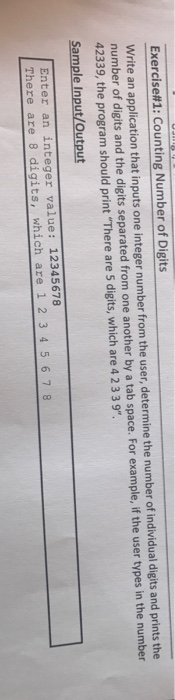Write an application that inputs one integer number from the user, determine the number of individual digits and print the number of digits and the digits separated from one another by a tab space. For example, if the user types in the 42339, the program should print “There are 5 digits, which are 4 2 3 3 9”. Enter an integer value 12345678 There are 8 digits, which are 1 2 3 4 5 6 7 8